# Image Manipulations in Python OpenCV (Part 1)

Published  January 15, 2019   0
M
Author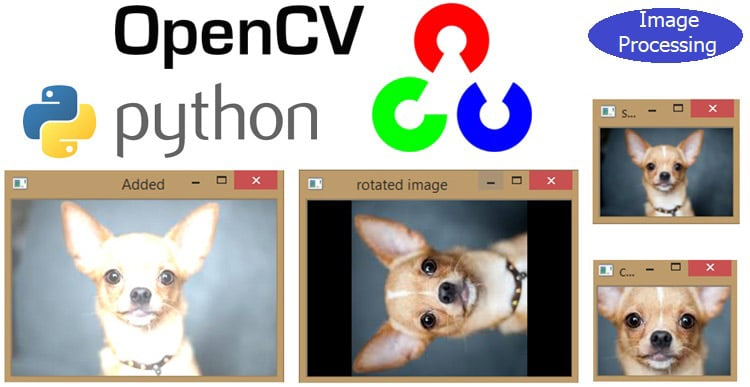In the previous tutorial, we have learned about OpenCV and done some basic image processing using it like grey scaling, color saturation, histogram, color spaces, RGB component etc. As told in the previous tutorial, OpenCV is Open Source Commuter Vision Library which has C++, Python and Java interfaces and supports Windows, Linux, Mac OS, iOS and Android. So it can be easily installed in Raspberry Pi with Python and Linux environment. And Raspberry Pi with OpenCV and attached camera can be used to create many real-time image processing applications like Face detection, face lock, object tracking, car number plate detection, Home security system etc.

In this tutorial, we are going to see how we are going to manipulate the image using OpenCV. Here we will learn to apply the following function on an image using OpenCV:

• Image Transformations – Affine and Non-Affine Transformation
• Image Translations – Moving image up, down, left and right
• Rotation of image – Spinning the image
• Scaling, Resizing, and Interpolation
• Image Pyramids – Another way of resizing
• Cropping – Cutting out the image region you want
• Arithmetic operations for Brightening and Darkening of images

### 1. Image Transformations – Affine and Non-Affine Transformation

Transformations are geometric distortions enacted upon an image, distortions certainly here not mean mistakes but a correction type to correct the perspective issues arising from the point where the image was captured. There are two Types of image transformations – Affine and Non-Affine

Affine transformations are of three types scaling, rotation and translation, the important thing in affine transformations is that lines are parallel before and after image transformations.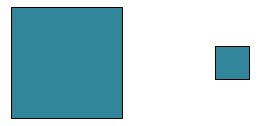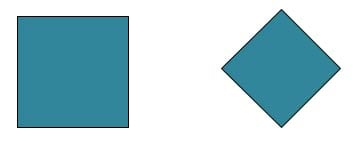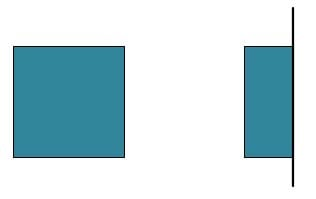Non-Affine transformations or projective transformations doesn’t preserve parallelism, length or angle, it does however preserves the collinearity and incidence, collinearity means that the two points lie on the same straight line.

Non-Affine transformations are very much common in computer vision and are generated from different camera angles. Non-affine or projective transformations are also called homography.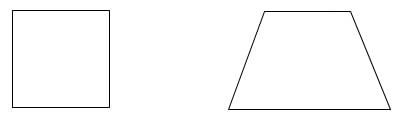### 2. Image Translations – Moving image up, down, left and right

Image translation is moving the image up, down, left and right and even diagonal if we implement x and y translation at the same time.

Now for performing image translations we use opencv’s warpAffine function, cv2.warpAffine is used to implement these translations but for that we need a translation matrix.

Translation matrix,

T = 1     0     Tx

0     1     ty

TX, Ty are the directions in which the image shifts takes place.

Wherein the TX is shift along X-axis (Horizontal)

TY is shift along Y-axis (Vertical)

```# this is an affine transformation that simply shifts the position of an image
# we use cv2.warpAffine to implement these transformations.
import cv2
import numpy as np
# store the height and width of image
height,width = image.shape[:2]
print(image.shape[:2])
quater_height, quater_width = height/4, width/4
T = np.float32([[1,0,quater_width],[0,1,quater_height]])
img_translation=cv2.warpAffine(image,T,(width,height))
print(T)
cv2.imshow('original_image', image)
cv2.waitKey(0)
cv2.imshow('Translation',img_translation)
cv2.waitKey(0)
cv2.destroyAllWindows()```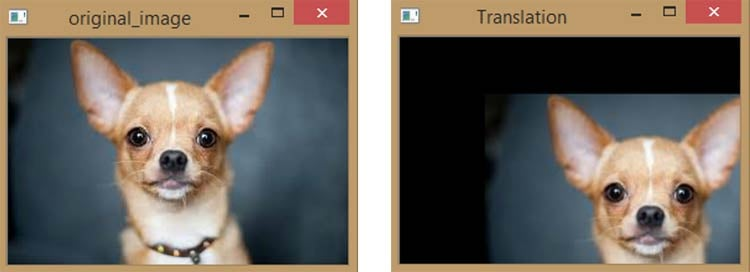Console Output - (183, 275) – height and width

[[ 1.    0.   68.75]     - T matrix

[ 0.    1.   45.75]]

### 3. Rotation of image – Spinning the image

Rotation of the image is rotating an image about a point or the point in the center of the image, just as the rotating point acts like a pivot.

As in translation we have T matrix, likely in rotation we have M matrix

Rotation matrix, M matrix = Cosθ             -Sinθ

Sinθ               Cosθ

Where the θ is the angle of rotation, measured in anti-clockwise direction.

Also there is one thing to note that OpenCV allows you to sale and rotate image at the same time using the function, cv2.getRotationMatrix2D(rotation_center_x, rotation_center_y, angle of  rotation, scale)

We still use opencv’s warpAffine function to get the rotation of the image but instead of translation matrix as in previous case here we use the rotation matrix.

```import cv2
import numpy as np
height, width=image.shape[:2]
#divide the height and width by 2 to rotate the image about it's center
rotation_matrix=cv2.getRotationMatrix2D((width/2, height/2),90,1)
rotated_image=cv2.warpAffine(image,rotation_matrix,(width,height))
cv2.imshow('original image',image)
cv2.waitKey(0)
cv2.imshow('rotated image', rotated_image)
cv2.waitKey(0)
cv2.destroyAllWindows() ```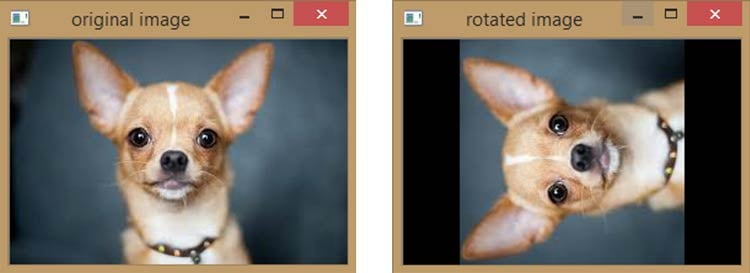Now the image is rotated by 90 degrees, it is cropped because of the canvas size, since the canvas size remains the same but due to rotation image size doesn’t fits into canvas size. It could be adjusted by setting the scaling factor to negative, but it allows a black background behind the image.

So you can set the height and width of image by anticipating it or guessing it or there is another method of rotating the image is by transposing it, but it would rotate the image by the multiples of 90 degrees in anti-clockwise direction.### 4. Scaling, Resizing and Interpolation

Scaling and resizing are affine transformations, resizing the image is what we have done quite a time and we have also dealt with interpolation, like when you are resizing the image to larger size in which we are expanding the pixels, there are some gaps in the pixels and that’s where interpolation comes in.

It can occur on increasing the image size from smaller to larger or decreasing the image size from larger to smaller.

Technically, interpolation is a method of constructing new data points (pixels), within discrete set of known data points.

There are various types of interpolation methods in OpenCV like

cv2.INTER_AREA – good for shrinking or down sampling

cv2.INTER_NEAREST - fastest

cv2.LINEAR – good for zooming or up sampling (default)

cv2.CUBIC - better

cv2.INTER_LANCZOS4 - best

```# resizing is very easy using the cv2.resize function, its arguments are
#cv2.resize(image,dsize(output image size), x_scale, y_scale, interpolation)
import cv2
import numpy as np
cv2.imshow('Original_image',image)
cv2.waitKey(0)
#let's make the image 3/4 the the original image size i.e. scales down to 75%
image_scaled=cv2.resize(image,None,fx=0.75,fy=0.75)
#since linear interpolation is default method for open cv we don’t need to implement it as a function.
cv2.imshow('scaling_linear interpolation', image_scaled)
cv2.waitKey(0)
#let's double the size of our image
img_double=cv2.resize(image,None,fx=2,fy=2,interpolation=cv2.INTER_CUBIC)
cv2.imshow('scaing_cubicInterpolation',img_double)
cv2.waitKey(0)
# let's do the resizing by exact dimensions
image_resize=cv2.resize(image,(200,300),interpolation=cv2.INTER_AREA)
cv2.imshow('scaling_exact',image_resize)
cv2.waitKey(0)
cv2.destroyAllWindows()```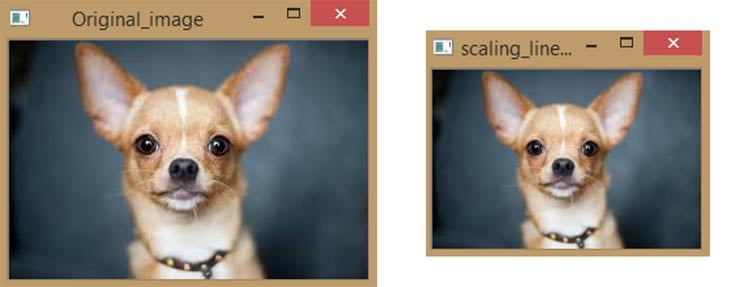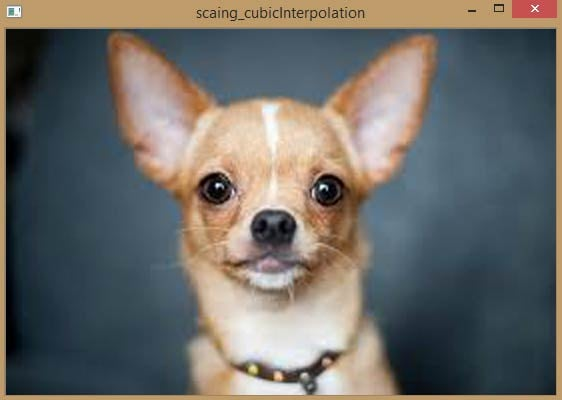### 5. Image Pyramids – Another way of resizing

Pyramiding image refers to either upscaling (enlarging images) or downscaling (shrinking images).

It’s simply a different way of resizing that allows us to easily and quickly scale images, scaling down reducing the height and width of the new image by half.

This comes useful when making object detectors that scales the images each time it looks for an object.

```import cv2
smaller=cv2.pyrDown(image)
larger=cv2.pyrUp(smaller)
cv2.imshow('original',image)
cv2.waitKey(0)
cv2.imshow('smaller',smaller)
cv2.waitKey(0)
cv2.imshow('larger',larger)
cv2.waitKey(0)
cv2.destroyAllWindows()```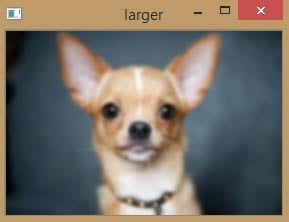In larger image you will notice that still being of the same size of the original image its little blurry because its being converted from smaller image to larger image directly. But if we interpolate it the image quality becomes improved as of the previous one because interpolation estimates the pixels while filling in the spaces when image is enlarged.

Now running the same code but with cubic interpolation gives a better quality of large image. Below images shows the comparison among original image, up scaled version of image, smaller image and cubic interpolated version of smaller image.

```import cv2
smaller=cv2.pyrDown(image)
larger=cv2.pyrUp(smaller)
cv2.imshow('original',image)
cv2.waitKey(0)
cv2.imshow('smaller',smaller)
cv2.waitKey(0)
cv2.imshow('larger',larger)
cv2.waitKey(0)
# increasing the quality of converted larger image from smaller image using cubic interpolation
img_double=cv2.resize(smaller,None,fx=2,fy=2,interpolation=cv2.INTER_CUBIC)
cv2.imshow('scaing_cubicInterpolation',img_double)
cv2.waitKey(0)
cv2.destroyAllWindows()```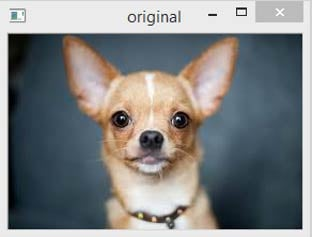NOTE: If you want to create multiple small copies of images going smaller and smaller in size or multiple large copies of images continuously growing in size, then we can use for loops or while loops keeping inside pyrDown or pyrUp function.

### 6. Cropping – Cutting out the image region you want

Cropping images refers to extracting a segment of image.

OpenCV directly don’t have cropping function but it can be easily done by numpy using the below code

`Cropped=image [start_row: end_row, start_col: end_col]`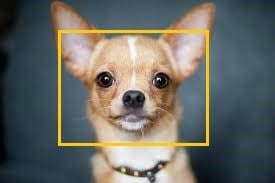We put the image array and using indexing tools or method in numpy, we define start row to end row and start column to end column separated by a comma that extracts the rectangle we want to crop to get the image.

```import cv2
import numpy as np
height, width=image.shape[:2]
#let's get the starting pixel coordinates(top left of cropping rectangle)
start_row, start_col=int(height*.25),int(width*.25)
#let's get ending pixel coordinates(bottom right)
end_row, end_col=int(height*.75),int(width*.75)
#simply use indexing to crop out the rectangle we desire
cropped=image[start_row:end_row, start_col:end_col]
cv2.imshow("original image",image)
cv2.waitKey(0)
cv2.imshow("cropped image”, cropped)
cv2.waitKey(0)
cv2.destroyAllWindows()```Note you can use pixel values directly instead of start_col or start_row, they are just given to get the easy identification for the user.

### 7. Arithmetic operations for Brightening and Darkening of images

Arithmetic operations in OpenCV basically are adding or subtracting matrixes to the image, adding or subtracting matrixes has effect on increasing or decreasing of brightness.

So to add or subtract matrixes we have to create them and numpy has a function called as np.ones that gives matrixes of 1’s same size as of our image.

```import cv2
import numpy as np
#create a matrix of one's, then multiply it by a scaler of 100'
#np.ones gives a matrix with same dimension as of our image with all the values being 100 in this case
M = np.ones(image.shape, dtype="uint8") * 100
#we use this to add this matrix M to our image
#notice the increase in brightness
cv2.waitKey(0)
#likewise we can also substract
#notice the decrease in brightness
subtracted=cv2.subtract(image,M)
cv2.imshow("subtracted",subtracted)
cv2.waitKey(0)
cv2.destroyAllWindows()```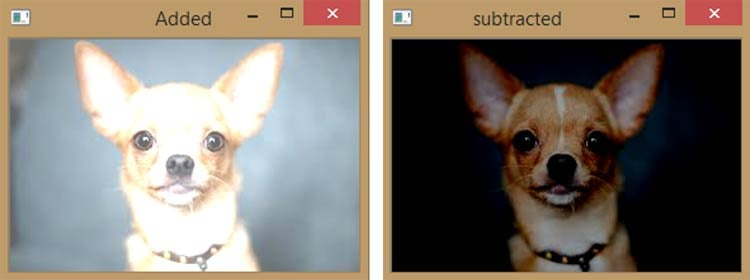This is how OpenCV can be used to apply many different image processing operations on image. We will continue with other image manipulation functions in next tutorial.